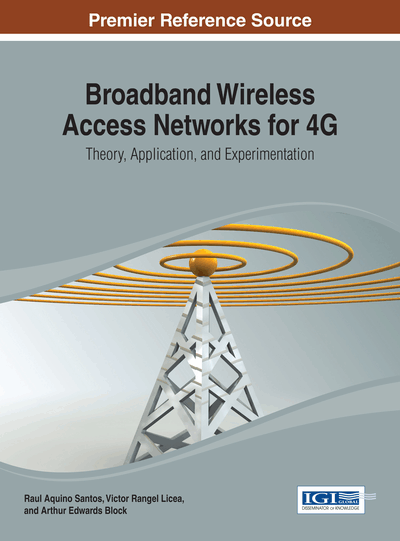Exploiting Scalar Effect in Wireless Access Networks: A Case Study and Research Directions

Rongrong Qian (Beijing University of Posts and Telecommunications (BUPT), China) and Yuan Qi (Beijing University of Posts and Telecommunications (BUPT), China)
DOI: 10.4018/978-1-4666-4888-3.ch009
Available
\$37.50
No Current Special Offers

Abstract

“Economies of scale” (called scale effect for notational convenience in this work), as a long run concept in microeconomics, refers to reductions in unit cost as the size of a facility and the usage levels of other inputs increase (Wikipedia, 2013). This chapter applies the concept of scale effect in microeconomics to the studies of algorithmic complexity within which the scale effect can be defined as the feature that the unit complexity (i.e., complexity per instance) of the algorithm decreases as the number of instances of algorithm increases while maintaining the performance. In this chapter, the Early-Termination Fixed-Complexity Sphere Detector (ET-FSD) is developed to exploit scale effect for the multiple-antenna system, which has to detect signals of multiple users under the constraint of sum complexity (e.g., the base-station systems always encounter the run-time limit of signal detection of all the users). Based on the study of ET-FSD, future directions of scale effect research for algorithmic complexity issues appearing in wireless access networks are presented as well.
Chapter Preview
Top

Introduction

The fundamental scenario for exploiting the scale effect (as shown in the Figure 1) is that some algorithm is instantiated for many times and all the instances are executed together under a sum complexity constraint. This algorithm shall have variable complexity, which has many parameters affecting the statistics of its complexity. It is assumed that the algorithm has instances, and the complexity of different instances (each of which is denoted by ξj) are random variables. Then, the sum complexity of instances is.

Due to the run-time limit, the sum complexity Sn cannot exceed a constraint Cn, otherwise, it leads to an outage event.

Considering the multiple-user scenario, it is proposed in this chapter to realize the full diversity multiple-input multiple-output (MIMO) detection under a polynomial constraint of unit complexity (i.e., complexity per user) by exploiting the scale effect. It is worth noting that the multiple-user scenario is a typical case of applying the scale effect, in which every algorithm is always instantiated for all the users. To be specific, the ET-FSD algorithm is developed in the multiple-user scenario, and the corresponding mathematic model primarily based on the large deviation principle is established for analyzing the scale effect. The existence of scale effect in ET-FSD can be clearly revealed by this study. Hence, one can expect that, due to the scale effect, the goal to make the MIMO detection work under a polynomial constraint of unit complexity while maintaining the full diversity performance can be accomplished.

After presenting ET-FSD as a case study, this chapter also focuses on discussing the future research directions of the scale effect study with respect to the algorithmic complexity issues in wireless access networks. Besides the MIMO detection, channel decoding algorithms (including these of Turbo codes and LDPC codes) of baseband processing in wireless access networks shall also have the scale effect. Thus this chapter will explain on the details of investigating the scale effect of channel decoding algorithms.

Top

Background

For the multiple-antenna system with nT transmit and nR receive antennas, one can use the matrixto represent this MIMO channel. The channel matrix considered in this study has the entries which are independent and identically distributed (i.i.d.) zero-mean complex Gaussian random variables with unit variance. If lettingbe the transmit signal vector in which each entry belongs to a constellation setwith size m, the signal model of the MIMO channel would be written as y=Hs+v, where denotes the receive signal vector and is the zero-mean circularly symmetric complex Gaussian noise vector with covariance matrix.

By assuming that is perfectly known at the receiver, the maximum-likelihood (ML) detector that could minimize the average error probability, is the optimal MIMO detection scheme and can be given by

(1)

The optimality of ML detector also implies that this detector is able to achieve the diversity order nR (i.e., full diversity order) (Paulraj, Nabar, &Gore, 2003). Despite the optimal performance of average error probability, the ML detector is not a feasible scheme of MIMO detection for practical deployment because of its extremely high complexity. It can be found from (1) that the ML detector has to perform exhaustive search among all the possible candidates and thus has the exponential complexity.

Complete Chapter List

Search this Book:
Reset